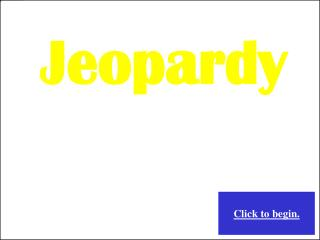DownloadDownload PresentationChoose a category. You will be given the answer. You must give the correct question.

# Choose a category. You will be given the answer. You must give the correct question.

Télécharger la présentation## Choose a category. You will be given the answer. You must give the correct question.

- - - - - - - - - - - - - - - - - - - - - - - - - - - E N D - - - - - - - - - - - - - - - - - - - - - - - - - - -
##### Presentation Transcript

1. Jeopardy Choose a category. You will be given the answer. You must give the correct question. Click to begin.

2. Name the Function Quadratic Formula Which form? Imaginary Numbers Factoring Parabolas \$100 \$100 \$100 \$100 \$100 \$100 \$200 \$200 \$200 \$200 \$200 \$200 \$300 \$300 \$300 \$300 \$300 \$300 \$400 \$400 \$400 \$400 \$400 \$400 \$500 \$500 \$500 \$500 \$500 \$500

3. A function represented by a line. Selection Board

4. What is a linear function? Selection Board

5. A function represented by a “V – shape” Selection Board

6. What is an absolute value function? Selection Board

7. A function represented by a half curve Selection Board

8. What is a radical function? Selection Board

9. A function represented by a parabola or “u-shape” Selection Board

10. What is a quadratic function? Selection Board

11. A function represented by an “s-shape” or snake Selection Board

12. What is a cubic function? Selection Board

13. The expression found underneath the radical in the formula. Selection Board

14. What is the discriminant? Selection Board

15. Points on the x-axis found by replacing y with zero in a quadratic equation. Selection Board

16. What are x-intercepts? Selection Board

17. The type of solutions found when simplifying the expression shown below Selection Board

18. What are complex numbers? Selection Board

19. The number of real solutions the graph of a quadratic equation associated with this solution will have. Selection Board

20. What is 2? Selection Board

21. The number of times the graph of a quadratic equation associated with this solution will touch the x-axis. Selection Board

22. What is once? Selection Board

23. A quadratic equation of the form , where a is a nonzero constant and the vertex of the graph is the point (h, k). Selection Board

24. What is vertex form? Selection Board

25. A quadratic equation of the form , where a is a nonzero constant and b and c are real numbers. Selection Board

26. What is standard form? Selection Board

27. The coefficient that remains the same in either form of a quadratic equation. Selection Board

28. What is a? Selection Board

29. The form that a quadratic must be written in before the quadratic formula can be applied. Selection Board

30. What is standard form? Selection Board

31. The form that allows readers to simply look at the function and know how it compares to the parent graph. Selection Board

32. What is vertex form? Selection Board

33. A symbolic representation equivalent to Selection Board

34. What is i? Selection Board

35. The real number obtained when multiplying i by itself. Selection Board

36. What is –1? Selection Board

37. The type of number that must appear underneath a radical for imaginary numbers to be considered. Selection Board

38. What is a negative number? Selection Board

39. Two numbers in the form a + bi and a – bi where a and b are real numbers. Selection Board

40. What are complex conjugates? Selection Board

41. For a fraction to be considered “in simplest form,” i cannot appear in this part of the fraction. Selection Board

42. What is the denominator? Selection Board

43. The process that must be completed first when factoring a 4-term polynomial. For example… Selection Board

44. What is grouping? Selection Board

45. The property that must be applied to solve a quadratic equation when one side is in factored form and the other side is zero. For example… Selection Board

46. What is the zero product property? Selection Board

47. The type of problem (emphasis on the operation) that you wish to create when rewriting a polynomial in factored form Selection Board

48. What is a multiplication problem? Selection Board

49. Given , these are the coefficients that you multiply together in an effort to factor a quadratic expression Selection Board

50. What are a and c? Selection Board